# Alkalmazott Analízis Szeminárium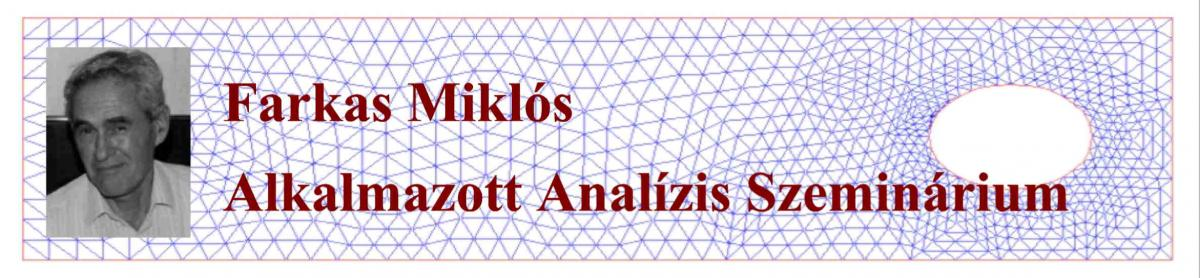A BME Matematika Intézet Analízis és Differenciálegyenletek Tanszékének közös Alkalmazott Analízis Szemináriuma 2016. őszén indult Faragó István (Differenciálegyenletek Tanszék) kezdeményezésére az MTA-ELTE Numerikus Analízis és Nagy Hálózatok Kutatócsoporttal együttműködésben. A szeminárium célja, hogy elősegítse egy alkalmazott analízissel (funkcionálanalízis, differenciálegyenletek, numerikus módszerek) foglalkozó kutatói kör kialakítását az intézeten belül. A szemináriummal fórumot szeretnénk biztosítani az alkalmazott analízissel foglalkozó matematikusok és az analízist alkalmazó kutatók számára az együtt gondolkodásra. További cél az érdeklődő hallgatók (MSc, PhD) bevonása a kutatói munkába.

Szemináriumunk 2017-től felvette a Farkas Miklós Alkalmazott Analízis Szeminárium nevet. Ezzel szeretnénk emléket állítani egyetemünk egykori tanszékvezető matematikaprofesszorának, aki elindította egyetemünkön a matematikus-mérnök képzést, és a stabilitáselmélet valamint a biomatematika terén elért jelentős tudományos eredményeivel ill. könyveivel nagyban hozzájárult az alkalmazott matematika erősödéséhez. (English version of the introduction.)

From the autumn semester of 2017 the talks will be in English on a regular basis. / 2017. őszi félévétől az előadásokat angol nyelven tartjuk.

Organizers / Szervezők:Faragó István1,2,3,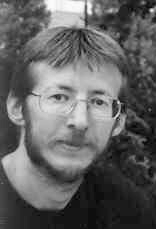Karátson János1,2,3 ,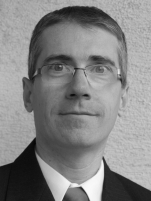Horváth Róbert1,3 ,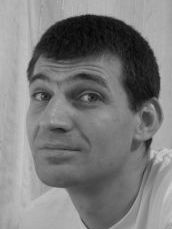Mincsovics Miklós1,3 ,Svantnerné Sebestyén Gabriella1,3 (1BME, 2ELTE, 3MTA-ELTE NUMNET)

Request for e-mail notifications and remarks to the organizers / Feliratkozás az e-mail listára ill. egyéb megjegyzések a szeminárium szervezőihez

Prospective speakers:

The talks of the Farkas Miklós Seminar are cancelled for the whole spring semester, owing to the special situation.

Next seminar:

We will continue in the autumn semester.

Previous seminars

5 March 2019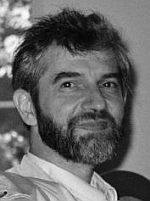Péter Surján (ELTE Institute of Chemistry, Laboratory of Theoretical Chemistry)

Coping with divergent perturbation series

Perturbation theory is a practically and conceptually important tool of physics, especially in quantum mechanics. It represents an ultimate method in the quantum chemical description of many-electron systems like atoms or molecules, where the Rayleigh-Schrödinger formulation is most frequently applied. In spite of 100 years of research, the sufficient and necessary conditions for the convergence of this infinite series is yet unknown. Even worse, the perturbation series is quite often divergent in many important applications.

After a short review on perturbation theory, we discuss some possibilities to accelerate convergence as well as some methods of resumming divergent series by means of complex analysis techniques. The latter include analytic continuation and the numerical solution of the inverse boundary problem, where the boundary values are sought for a partial differential equation on the complex plane in the knowledge of the solution of that equation in certain domain.

27 February 2019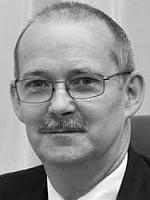Zsolt Páles (University of Debrecen, Institute of Mathematics)

Solution methods for two variable functional inequalities

In this talk we consider two classes of functional inequalities and the related functional equation. Under natural but general circumstances we show that if the solution set of the functional equation forms a 2-dimensional Beckenbach family then the continuous solutions of the functional inequality are exactly those functions which are convex with respect to the Beckenbach family. Several concrete examples and applications will be provided.

20 February 2019Mihály Hujter (BME, Department of Differential Equations)

Miklós Farkas and a brief history of applied math at TU Budapest

The history of the Technical University in Budapest began in the 18th century. The deep mathematical studies started around 1840. Among others, VállasHunyady, J. Kőnig, Rados, Kürschák, D. Kőnig, Egerváry, Hajós, Gallai, Alexits were important professors of math.

For more than 50 years Miklós Farkas (1932-2007) was a dominant person in applied mathematics education, mainly for mechanical engineering students. He published (wrote and edited) 15 books and 77 papers. The most famous is ,,Periodic Motions'' (Berlin, 1994).

Visit the ,,História Tudósnaptár'' homepage for more details on the above listed persons: https://tudosnaptar.kfki.hu/historia/

5 December 2019Research reports of PhD students

10:15-10:35: Lívia Boda: Investigation of different operator splitting methods

10:35-10:55: András Molnár: Adaptive Time-Stepping for Neural Ordinary Differential Equations

10:55-11:15: Teshome Bayleyegn: Absolute stability and convergence analysis for Multiple Richardson Extrapolation

28 November 2019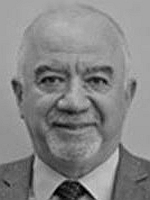Ivan Dimov (Institute of Information and Communication Technologies, Bulgarian Academy of Sciences)

Multidimensional Sensitivity Analysis of Large-scale Mathematical Models

The aim of the talk is to present various approximation Sensitivity Analysis techniques of Large-scale Mathematical Models. A concept of Sensitivity Analysis and complexity in classes of algorithms will be presented. More precisely, randomized Quasi-Monte Carlo and modified Sobol sequences will be analyzed. As a case study the Unified Danish Eulerian model of air pollution transport (UNI-DEM) will be considered. UNI-DEM is carried out to compute more precisely interaction effects of inputs and sensitivity measures. Various Monte Carlo algorithms have been applied for numerical integration of multidimensional integrals to estimate the sensitivity measures. A comparison of their efficiency will be discussed.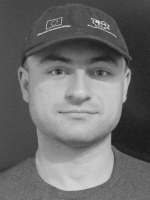Venelin Todorov (Institute of Information and Communication Technologies, Bulgarian Academy of Sciences)

High-accuracy numerical methods for parabolic systems in air pollution modeling

We present different approach es for enhancing the accuracy of the second-order finite difference approximations of two dimensional semilinear parabolic systems. These are the fourth-order compact difference scheme and the fourth-order scheme based on Richardson extrapolation. Our interest is concentrated on a system of ten parabolic partial differential equations in air pollution modeling. We analyze numerical experiments to compare the two approaches with respect to accuracy, computational complexity, nonnegativity preserving, etc. The sixth-order approximation based on the fourth order compact difference scheme combined with Richardson extrapolation is also discussed numerically.
(Joint work with Juri Kandilarov, Ivan Dimov and Luben Vulkov.)

21 November 2019

Chemical Resonance and Beats in Periodically Forced Chemical Oscillatory Systems

We present the existence of resonance and beats in open and forced chemical oscillatory systems using a superimposed sinusoidal modulation on the inflow rates of the reagents. We demonstrate control over the periodicity of the forced oscillations and show that the time period of beats follows the relation known for forced physical oscillators. Based on experimental results and numerical model simulations, we could show that resonance and beats are internal properties of chemical oscillatory systems. A forced open chemical oscillatory system is a counterpart of the forced oscillators known form the classical mechanics (e.g., driven pendulum), in which instead of applying a periodic external driving force, the periodically changing chemical potential drives the open oscillatory systems.
(joint work with Hugh Shearer LawsonGábor Holló, Róbert Horváth)

14 November 2019Christian Pötzsche  (Department of Mathematics, Alpen-Adria-University Klagenfurt, Austria

Bifurcation and Discretization in Integrodifference Equations

Integrodifference equations are infinite-dimensional dynamical systems in discrete time. They are motivated by theoretical ecology in order to describe the spatial dispersal and temporal evolution of species having non-overlapping generations. In this talk, we review some recent work addressing two aspects concerning their long-term behavior:
(1) Bifurcation theory of periodic equations, which requires a combination of analytical and numerical techniques (joint work with Christian Aarset)
(2) Numerical Dynamics (persistence of dynamical properties under numerical discretization)

7 November 2019Gabriella Vas (MTA-SZTE Analysis and Stochastics Research Group, Bolyai Institute, University of Szeged)

Large-amplitude periodic orbits for delay equations

Let us consider scalar delay differential equations of the form x'(t)=-ax(t)+f(x(t-1)), where a>0 and f is a nondecreasing C1-function. This talks gives an overview of the periodic orbits and the global attractor.

After showing some well-known results of Tibor Krisztin, Hans-Otto Walther and Jianhong Wu, I introduce the notion of large-amplitude periodic (LAP) orbits. First we discuss the bifurcation and the existence of a pair of LAP orbits. Then we describe the geometric properties of the unstable set of a specific LAP orbit in detail. Complicated configurations of LAP orbits appear when the dynamical system has several unstable equilibria – we also consider this case. These are joint works with Tibor Krisztin and Szandra Beretka.

No preliminary knowledge of delay equations is presumed.

24 October 2019Svetozar Margenov (Institute of Information and Communication Technologies, Bulgarian Academy of Sciences)

Numerical Solution of Fractional Diffusion Problems

This study is motivated by the recent achievements in fractional calculus and its numerous applications related to anomalous (super) diffusion. Let us consider a fractional power of a self-adjoint elliptic operator introduced through its spectral decomposition. It is self-adjoint but nonlocal. The nonlocal problems are computationally expensive. Several different techniques were recently proposed to localize the nonlocal elliptic operator, thus increasing the space dimension of the original computational domain.

An alternative approach [1,2,3] is discussed in this talk. Let $\cal A$ be a properly scaled symmetric and positive definite (SPD) sparse  matrix, arising from finite element or finite difference discretization of the initial (standard, local) diffusion problem. A method for solving algebraic systems of linear equations involving $\cal A^\alpha$, $0 < \alpha < 1$, is presented. The solution methods are based on best uniform rational approximations (BURA) of the scalar function $t^{\alpha}$, $0\le t\le 1$. The method has exponential convergence rate with respect to the degree of rational approximation $k$. The error estimates of the last variant of BURA methods are robust with respect to the spectral condition number $\kappa (\cal A)$. A stabilized modification of the Remez algorithm is developed to compute the BURA of $t^{\alpha}$. Although the fractional power of $\cal A$ is a dense matrix, the algorithm has complexity of order $O(N)$, where $N$ is the number of unknowns. At this point we assume that some solver of optimal complexity (say multigrid or multilevel) is used for the involved systems with matrices ${\cal A} + d_j \cal I$, $d_j \ge 0$, $j=1, \dots, k$. The comparative numerical tests confirm the advantages of the BURA method.

Acknowledgement: This research has been partially supported by the Bulgarian NSF Grant DN12/1.

17 October 2019László Székelyhidi (Universität Leipzig)

Renormalized and non-renormalized solutions of the transport equation

The linear transport equation is the possibly simplest of all PDE, describing the evolution of a density under the flow of a given vectorfield.
It is well-known that, as long as the vectorfield is Lipschitz continuous,  solutions of the linear transport equation are closely related, via the method of characteristics, to the Lagrangian flow map generated by the vectorfield. However, this link breaks down if the vectorfield is merely Sobolev, since in this case the ODE does not make sense classically. Of course, formulation of the PDE poses no problems even without differentiability assumptions. On the other hand there are numerous applications in fluid mechanics and kinetic theory, where the transport equation appears with a vectorfield which is in some Sobolev space, possibly even continuous, but not Lipschitz. For such cases DiPerna and Lions developed in the late 1980s a theory of renormalization, leading to a well-posed solution concept for both the PDE and the ODE. In the talk we discuss the limits of this theory and present examples showing that unless certain additional integrability conditions are imposed, the theory of renormalization does not lead to a unique solution.

10 October 2019Tamás Kalmár-Nagy (BME, Department of Fluid Mechanics)

Having fun on the plane: Poincaré-Lyapunov constants, Jacobians, Quadrics and Jordan Forms​

In nonlinear dynamical system self-excited vibrations frequently occur where an equilibrium undergoes a Hopf bifurcation and limit-cycle oscillations develop. The Hopf bifurcation has two types, supercritical (soft) and subcritical (dangerous). The type of the bifurcation depends on whether the nonhyperbolic equilibrium is weakly stable or unstable. The stability of the equilibrium (and thus the type of the Hopf bifurcation) is determined by the sign of the so-called Poincaré-Lyapunov constant. This talk discusses three short topics centered around Poincaré-Lyapunov constants:
1, We pose and affirmatively answer the question whether the stability of a nonlinear center can be determined from the eigenvalues of the Jacobian matrix AWAY from the equilibrium point.
2, We recognize that the Poincaré-Liapunov constant is a quadratic form in a 10-dimensional space of the coefficients associated with the normal form of a Hopf bifurcation. This real manifold (the "Hopf quadric") separates regions of the parameter space corresponding to supercritical and subcritical bifurcations. The stationary points of the squared distance function from a parameter point to the Hopf quadric are the real zeros of a univariate algebraic equation. The distance to the quadric is the minimal positive zero of this equation. This distance can be used as a measure of the "criticality" of the bifurcation. Joint work with Alexei Yu. Uteshev.
3, We use the so-called Carleman embedding technique to recast the normal form of a Hopf bifurcation as an infinite-dimensional linear system. We describe the connection between the Poincaré-Lyapunov constants and the linear algebraic properties of the Carleman matrices. This connection provides a new algorithm to compute Poincaré-Lyapunov constants. Joint work with Csanád Hubay.

3 October 2019Zahari Zlatev (Aarchus University, Roskilde, Denmark)

Using climatic scenarios in advanced air pollution studies

Systems of non-linear partial differential equations (PDEs) are often used to describe mathematically the long-range transport of air pollutants. The discretization of the spatial derivatives involved in these systems of PDEs leads to the solution of large systems of non-linear ordinary differential equations (ODEs), which are very stiff and, therefore, must be handled by applying implicit numerical methods for solving systems of ODEs. That leads to the solution of systems of non-linear algebraic equations, which have to be treated, at every time-step, by suitable iterative methods. Some version of the well-known Newton Iterative Method is normally used and systems of linear algebraic equations (LAEs) are to be solved many times in the inner loop of the Newton procedure. The systems of LAEs are huge when fine spatial resolution is used, which is nearly always highly desirable. Moreover, many such systems are to be treated, because the time-interval is nearly always very long. Handling many millions of systems of LAE’s, each of which contain several hundred million equations, is not unusual. Therefore, such complex models have necessarily to be run on high-performance computers by applying special techniques; see, for example, Z. Zlatev and I. Dimov: “Computational and Numerical Challenges in Environmental Modelling”, Studies in Computational Mathematics, Vol. 13, Elsevier, Amsterdam, 2006. The problems are becoming much more difficult and time-consuming when large-scale air pollution models (a) are used to study the sensitivity of the pollution levels to variations of some key parameters as, for example, the emissions and (b) are combined with different climatic scenarios in the efforts to investigate the influence of climatic changes on some high and harmful pollution levels. The treatment of the air pollution models in this extremely difficult situation will be discussed in this talk. It will, furthermore, be shown that the climatic changes are normally resulting in increased levels of some pollutants. However, the major aim will be to demonstrate the fact that some of these enormous computational tasks cannot be handled directly even on the fastest parallel computers. Therefore, some special techniques, fast numerical methods and appropriate splitting procedures must necessarily be used.

26 September 2019Éva Gyurkovics (BME, Department of Differential Equations)

Stability and stabilization with applications

In this talk, a short survey will be given about the results of the last three years achieved by several colleagues. Firstly, the stability analysis of continuous- and discrete-time time-delay systems based on a set of Lyapunov–Krasovskii functionals (LKFs) will be discussed. An important task in this problem is the estimation of the derivatives and differences of the LKFs. To this end, new multiple integral and summation inequalities will be presented that involve several famous inequalities known before. It will be shown that the proposed set of sufficient stability conditions given by LMIs can be arranged into a bidirectional hierarchy establishing a rigorous theoretical basis for comparison of conservatism of the investigated methods. Sufficient stability conditions will also be presented for the case of time-varying delays based on a parameterized family of LKFs involving multiple integral terms. Comparisons of several bounding inequalities proposed recently for the estimation of integrals and sums of quadratic functions will be discussed: the equivalences of several known variants of the free matrix based inequalities and their generalized and simplified forms are shown. Then, the relationship between the (simplified) free matrix based inequality and the combination of the Bessel-based inequality with different bounding inequalities affine in the length of the intervals are investigated.

Secondly, the results are applied to the non-fragile exponential synchronization problem of complex dynamical networks with time-varying coupling delays via sampled-data static output-feedback controller involving a constant signal transmission delay.

Finally, an algorithm terminating in finitely many steps will be given to determine the dynamic output feedback control with suboptimal finite-frequency H norm bound. Two case studies will be presented to illustrate the effectiveness of the proposed method.

19 September 2019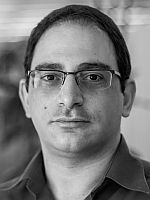Yiannis Hadjimichael (NUMNET MTA-ELTE research group)

High order discretization methods for spatial dependent SIR models

In this talk, an SIR model with spatial dependence is discussed and results regarding its stability and numerical approximation are presented. SIR models have been used to describe epidemic propagation phenomena, and one of the first models is derived by Kermack and McKendrick in 1927. In such models, the population is spit into three classes: $S$ is the group of species susceptible to infection, $I$ is the compartment of the ill species, and $R$ the class in of recovered species. We consider a generalization of the original Kermack and McKendrick model in which the size of the populations differs in space. The use of local spatial dependence yields a system of integro-differential equations. The uniqueness and qualitatively properties of the continuous model are analyzed. Furthermore, different choices of spatial and temporal discretizations are deployed, and step-size restrictions for population conservation, positivity and monotonicity preservation of the discrete model are investigated. We provide sufficient conditions under which high order numerical schemes preserve the discrete properties of the model. Computational experiments verify the convergence and accuracy of the numerical methods.

Presentations in 2018/192017/182016/17